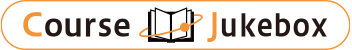### CourseDetailDegree
Bachelor
3
Course delivery methods
face-to-face
Subject
Physical sciences, Mathematical sciences, Engineering & technology
Program
School
School of Science and Engineering
Department
Bachelor's Program in Interdisciplinary Engineering
Campus
Tsukuba Campus
Classroom
3A408
Course Offering Year
2023-2024
Course Offering Month
October - December
Weekday and Period
Wed5
Capacity
Credits
1.0
Language
English
Course Number
FJ12101

### Statistical Physics I University of Tsukuba

#### Course Overview

Statistical Physics as well as Quantum Mechanics provides the most important backbone of modern physics. In the present course, the basic principles of statistical mechanics are explained. After reviewing the basics of probability theory, the fundamental assumption of Statistical Mechanics, "principle of equal a priori probabilities," is introduced to construct statistical ensembles. The microscopic interpretation of entropy is explained so that the connection to thermodynamics becomes constructed.

#### Learning Achievement

1. The students are able to calculate probabilities with the binomial, normal, and Poisson distributions.
2. The students are able to explain the difference of the mechanical energy and thermal energy.
3. The students are able to explain the microscopic and macroscopic parameters, reversibility and irreversibility of statistical systems.

#### Competence

Related to 2. Understanding ability of physical phenomena.

#### Course prerequisites

Intro. to Single-Variable Calculus I & II, Advanced Calculus, Linear Algebra I & II, Probability and Statistics, Mechanics, Electromagnetism, Thermodynamics, Modern Physics

Homework & Class performance 30 %
Final 70%

#### Course schedule

Random walk and statistical Mmethods
Probability distributions
Change of random variables 1
Change of random variables 2
Microscopic States versus Macroscopic states
The fundamental postulate: statistical (micro-canonical) ensemble:
Classical interpretation of microscopic states
Exact and inexact differentials
First law of thermodynamics
Fundamental equations and equations of states

Lectures